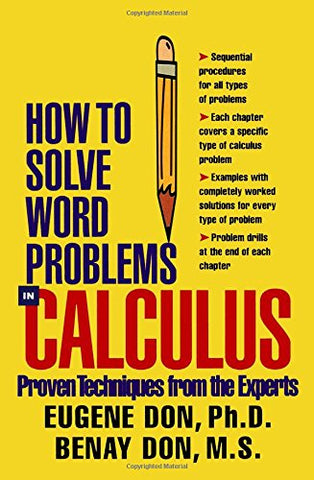# How to Solve Word Problems in Calculus

• ISBN-13: 9780071358972
• \$11.58

Only 3 left!

Considered to be the hardest mathematical problems to solve, word problems continue to terrify students across all math disciplines. This new title in the World Problems series demystifies these difficult problems once and for all by showing even the most math-phobic readers simple, step-by-step tips and techniques. How to Solve World Problems in Calculus reviews important concepts in calculus and provides solved problems and step-by-step solutions. Once students have mastered the basic approaches to solving calculus word problems, they will confidently apply these new mathematical principles to even the most challenging advanced problems. Each chapter features an introduction to a problem type, definitions, related theorems, and formulas. Topics range from vital pre-calculus review to traditional calculus first-course content. Sample problems with solutions and a 50-problem chapter are ideal for self-testing. Fully explained examples with step-by-step solutions.